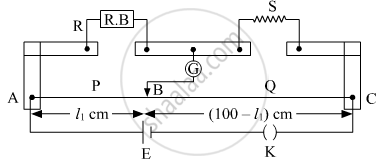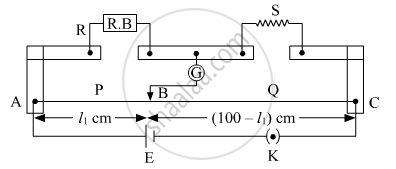# What is the End Error in a Meter Bridge? How is It Overcome? the Resistances in the Two Arms of the Metre Bridge Are R = ω and S Respectively. When the Resistance - Physics

#### Question

What is the end error in a meter bridge? How is it overcome? The resistances in the two arms of the metre bridge are R = Ω and S respectively.  When the resistance S is shunted with equal resistance, the new balance length found to be 1.5 l1, where l2 is the initial balancing length. calculate the value of s.#### Solution

The shifting of zero of the scale at different points as well as the stray resistance gives rise to the end error in meter bridge wire. This error arises due to the non-uniformity of the meter wire End corrections can be estimated by including known resistances P1 and Q1 in the two ends and finding the null point.

We haveR = 5 Ω

According to wheat stone bridge principle:

R/l_1 = S/(100 - l_1)

5/l_1 = S/(100 - l_1)    ....(1)

After shunting, connect the resistance in parallel

S → S/2

5/(1.5 l_1) = S/(2(100 - 1.5 l_1))  ....(2)

Thus, equation (1) can be written as

500 - 5l_1 = Sl_1                       ....(3)

And equation 2 is

10(100 - 1.5l_1) = 1.5 Sl_1       ....(4)

From equation (3) and (4)

(500 - 5l_1)/l_1 = (1000 - 15l_1)/(1.5l_1)

750 - 7.5l_1 = 1000 - 15l_1

-250 = -7.5l_1

l_1 = 100/3

S = (500 - (5 xx 100)/3)/(100/3) = (500 - 500/3)/(100/3) = 1000/3 xx 3/100

S = 10 Ω

Is there an error in this question or solution?